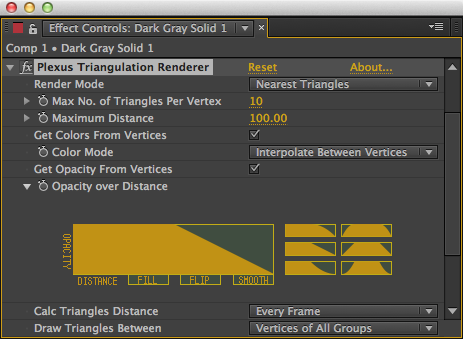## Triangulation Render ObjectTriangulates points using different modes to create triangles between points. Unlike the Facets, effectors can’t effect the triangles created by the triangulation object.

Render Mode: Set the current render mode:

• Nearest Triangles: Just like Nearest Lines, Nearest Triangles connects closest points.

• Outer Surface: It triangulates the Imaginary outer surface of a group of vertices.

• Mesh Triangulation: It triangulates the given vertices, as if it were a part of the mesh.

Maximum Distance: The Maximum Distance between three vertices until which the triangles are rendered.

Max No.of Triangles: The maximum number of triangles each vertex is connected to. Active only during the Nearest Triangles Render mode.

Get Colors From Vertices: If selected, the ‘Color’ parameter is overridden and the color is obtained from the vertices.

Color Mode: Sets the Color Interpolation mode between the vertices.

Get Opacity From Vertices: If selected the ‘Opacity’ parameter is overridden and the opacity is obtained from the vertices.

Opacity over Distance: Let’s you specify how the Opacity changes over distance.

Calc Lines Distance: You can choose between three modes.

• Every Frame: This option calculates the distance between the points every frame. It can be jittery sometimes.

• Only One Frame: Calculate the distance between the points only one frame and use that distance for all the frames.

• Over Time Interval: Calculate the distance between the points over a time interval and smoothly interpolates them.

Draw Triangles Between: Popup to Draw triangles from vertices in between the same group, or in between different groups or in between all the groups, or just two groups.